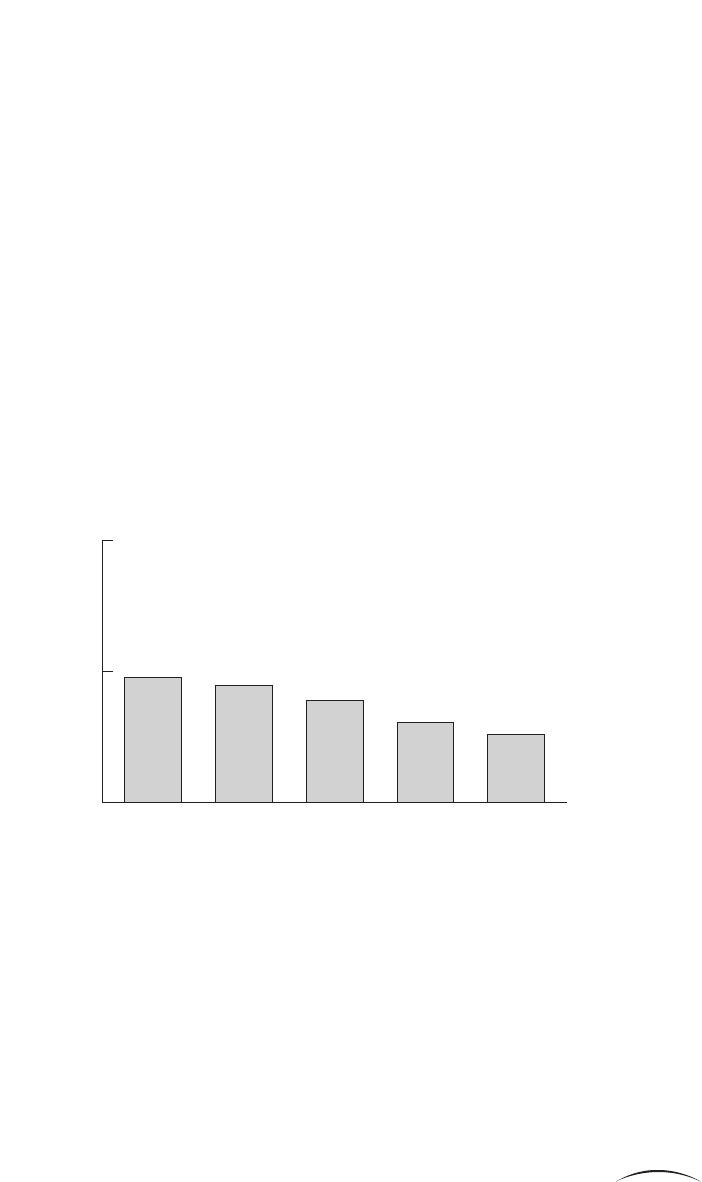a c c o u n t s d e m y s t i f i e d
156
Since operating profit is what we have left after paying the oper-
ating expenses, it obviously makes sense to analyse the operating
expenses and see if there is anything we can learn.
The capital employed in a business is made up of both fixed assets
and working capital. Working capital is, in turn, made up of vari-
ous different elements. We can therefore analyse each of these
different elements.
In the same way that we looked at how many sales we got for each pound
of assets and how much profit we got for each pound of sales, we will
analyse all the expenses and the constituents of capital employed in rela-
tion to sales.
Expense ratios
The P&L lists three types of expense: cost of goods sold, distribution and
administration. Let’s look at these three first.
Cost of goods sold, gross margin
As you will remember, the cost of goods sold (‘COGS’) is exactly what it
says the cost of buying and/or making the goods to be sold. From the
P&L, we know that Wingate’s cost of goods sold in year five was £8,078k.
We can therefore divide this by the sales of £10,437k to give us cost of
goods sold as a percentage of sales (‘COGS%’).
COGS% = COGS / Sales
= 8,078 / 10,437
= 77.4%
We also saw earlier that the profit left after subtracting cost of goods sold
from sales is known as gross profit. Gross profit as a percentage of sales is
known as gross margin.
Gross margin = Gross profit / Sales
= 2,359 / 10,437
= 22.6%a n a ly s i s o f t h e e n t e r p r i s e
157
Gross margin and the cost of goods sold percentage effectively tell you the
same thing. You will find that most people talk about gross margin.
How does this relate to ‘mark-up’ then, Chris?
If you have something that cost you 77.4 pence and you sell it for £1, your
cost of goods is 77.4 per cent and your gross margin is 22.6 per cent, just
as we have seen for Wingate. Mark-up is the percentage that you add on
to your cost to get your selling price, which we calculate by dividing your
gross profit by your cost:
Mark-up = Gross profit/Cost
= 22.6 / 77.4
= 29.2%
Let’s now look at how Wingate’s gross margin has changed over the last
five years [Figure 9.4].
As you can see, there has been a steady decline in gross margin.
So what this says is that every year a pound’s worth of sales is costing us more to pro-
duce than the previous year?
Yes, but the way you phrase it makes it sound as if the fault must lie with
the director in charge of manufacturing. If the factory is badly run, then
you may well be right, but there is an alternative explanation.
What you have to remember with all these analyses is that sales value
(i.e. the figure for sales in the accounts) is made up of sales volume
Figure 9.4 Wingate’s gross margin
20%
24.8%
24.5%
23.9%
23.1%
Yr 1 Yr 2 Yr 3 Yr 4 Yr 5
22.6%
25%
30%

Get Accounts Demystified, 6th Edition now with the O’Reilly learning platform.

O’Reilly members experience live online training, plus books, videos, and digital content from nearly 200 publishers.